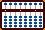The Bead Unbaffled - An Abacus Manual

###Negative Temperatures

Negative Number Conundrum. (If the two techniques multiplying negative numbers and negative numbers have not been fully understood, this exercise may be confusing.)

Because of my proximity to the US/Canadian boarder and depending on what radio/television stations I'm listening to, I get weather reports in both Celsius and Fahrenheit. I need a quick and simple formula to calculate back and forth. When calculating positive temperatures the formula is very straight forward and easy to follow.

For example: To change Celsius to Fahrenheit I've learned the following simple formula. It's perfectly accurate:

Celsius *2 -10% + 32 = Fahrenheit

Working the other way is slightly less precise because  **11% should actually be 11.111 ==> **  Nonetheless the formula is accurate enough to be extremely close. To change Fahrenheit to Celsius the formula is:

Fahrenheit - 32 +11% /2 = Celsius

When I use the above formulas with negative numbers (winter time temperatures) the process gets a little more challenging and really fun.

Example 1: Convert -6 Celsius to Fahrenheit

The formula is -6 *2 -10% +32 = 21.2

Place -06 on the soroban (the actual beads on the soroban read 94).

-06 *2 = -12. In this case, the quickest way to multiply by 2 is subtract a further 6. In order to do this we need to borrow. Borrow 1 from 9 on the left and subtract a further 6 to equal -12 (the actual beads on the soroban now read 88).

Subtract 10% from -12. **Two negatives multiplied together equal a positive so instead of subtracting we have to add 10%.**  Add 1.2 to 88. The negative (complementary) number now shows -10.8 (the actual beads on the soroban read 89.2).

Now add 32.   89.2 + 32 = 121.2  **Because we've now reached a positive number, give back 100 (the amount borrowed in the above step)**  The soroban beads now read the positive number 21.2, which is the answer.

Example 2: Convert 14 Fahrenheit to Celsius

The formula is 14 - 32 +11% /2 = -9.99

Place 14 on the soroban

14 - 32 = -18. **In order to do the following we need to borrow from the rod on the left. Subtract 32 from 14 to equal -18 (the actual beads on the soroban read 82).

Add 11% to -18. **A negative multiplied by a positive equals a negative so we have to subtract.** Subtract 11% from 82. **A reasonably simple and quick way to subtract 11% is to move one rod to the right, subtract 18 then move one more rod to the right and subtract a further 18.** (see below)

A B C D
8 2 0 0
- 1 8
- 1 8

8 0 0 2 ==>
The negative number now shows -19.98 while the actual beads show 80.02

Initially this is where I had trouble. The solution for me was; before continuing return the amount borrowed when subtracting 32 from 14. In other words, add 1 to the rod on the left. The beads on the soroban read 18002.

Divide 18002 by 2.  The result leaves the beads on the soroban reading 9001 while the negative (complementary) number shows 0999 or -9.99, which is the answer.